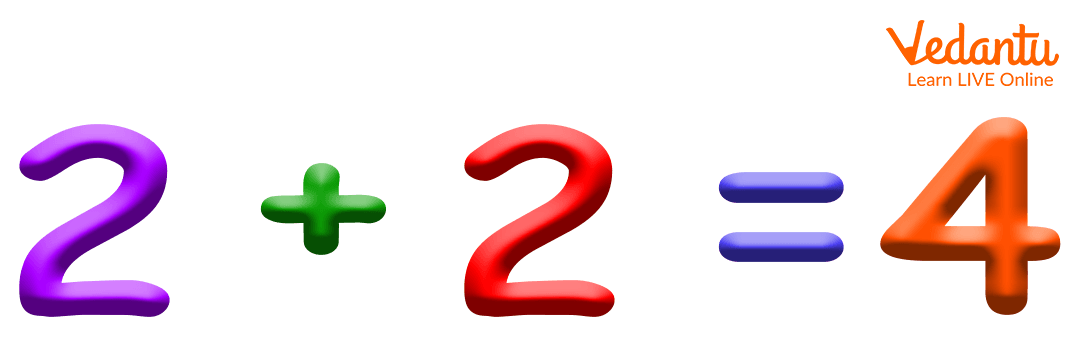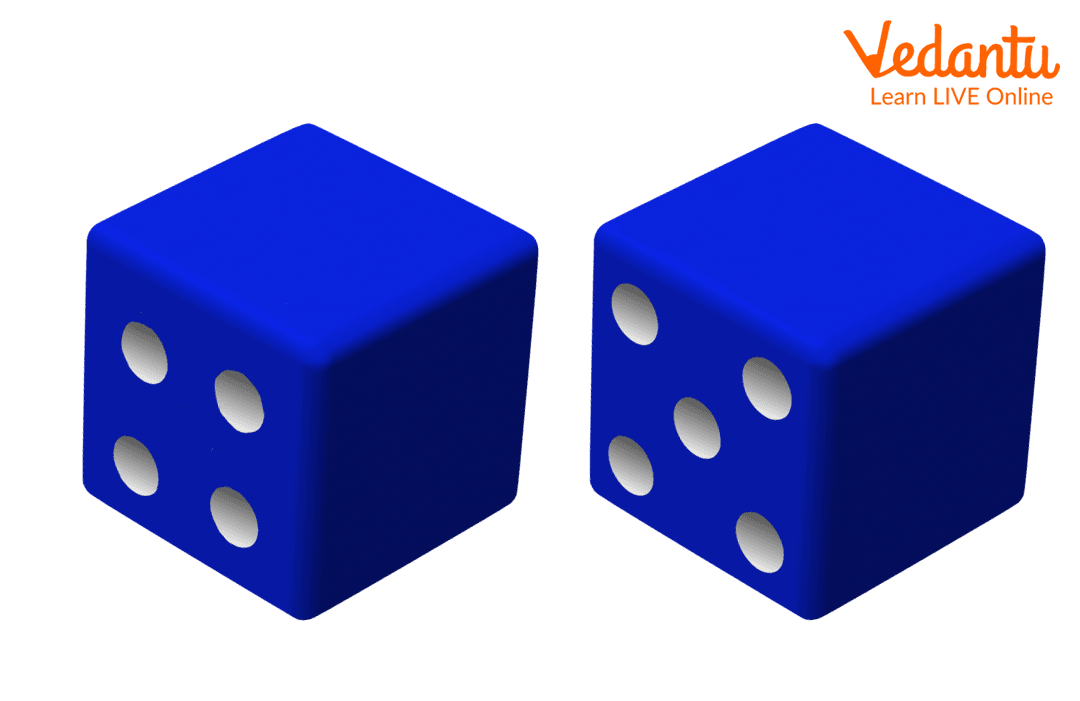Courses
Courses for Kids
Free study material
Free LIVE classes
More

# Addition & Subtraction for 1 Year Kids (Age Group of 5-6)## An Introduction to Addition & Subtraction in Year 1 for Kids

Kids of age 5-6 years start reading and writing. This is the right time when we can teach them the mathematical operations of addition and subtractions. We can introduce them to these concepts with some tricks so that kids not only follow the steps of addition and subtraction but also get an idea of what happens during these operations. In this way, they can practise counting and start solving simple word problems pretty soon. In this article, parents and teachers will get some tricks and ideas for teaching addition and subtraction in year 1 for kids.

## Ideas of Teaching Addition and Subtraction in Year 1

There are ideas that can help our child to understand addition and subtraction. A few ideas are discussed below.

1. ### Play the Number Facts Pairs Game

You can also tell your child a range of number facts involving addition and subtraction. Number facts are facts that are memorised without the need to calculate, for example, 2 + 2 is equal to 4. It can be introduced as a fact to your child. There is a lot of fun playing such games with kids. They will quickly recall fast some small calculations without doing any written calculations.Number Facts

1. ### Play Dice Games

This is a fun game to encourage children to solve addition and subtraction problems practically. To play this game we all need two dice. We may play a game of two dice where each player rolls two dice and next has to find the total. The winner is the player who has the largest total. We can play similar games for subtraction where subtraction is performed and again who has the highest total will be the winner. It will help kids to do calculations fast.Double Dice Game

1. ### Subtraction as Difference

We should teach our child subtraction as ‘difference’. For example:’12 – 2′ can also be thought of as ‘What is the difference between 12 and 2?’ We teach subtraction by showing them the difference between objects. For example, we have a group of toys and then we remove some toys and then ask them to count the difference. This will help kids not only to do subtraction but also to understand the real meaning of subtraction.

1. ### Focus on Counting of Objects

Kids learn addition and subtraction through the counting of objects easily. For example, if you have 8 apples and your kid has 4 apples. You can ask her or him the total number of apples. Your child will count each apple one by one and will give you the right answer doing so. Similarly, you can teach subtraction.

## Conclusion

The above article discusses some ways which will help kids to understand and learn the mathematical operation of addition and subtraction. It is important to teach kids mathematical concepts through games to make the learning experience fruitful. Including fun in your teaching will increase their interest in studies and ensure quick absorption of knowledge.

Last updated date: 21st Sep 2023
Total views: 136.2k
Views today: 3.36k

## FAQs on Addition & Subtraction for 1 Year Kids (Age Group of 5-6)

1. What are addition and subtraction?

Addition and subtraction are the two basic mathematical arithmetic operations. In addition, we add two numbers or objects and in subtraction, we find the difference between two or more numbers or objects or any mathematical values. The other two basic mathematical operations are multiplication and division. The sign of addition is '+' and subtraction is '-'.

2. How to teach a kid addition and subtraction?

When kids need to be introduced to the basic operations of Mathematics comprising addition and subtraction, they can be taught through the addition or subtraction of objects such as fruits, toys and any objects of surroundings. We should play games of addition and subtraction with children. Once you’ll make these concepts interesting to understand, kids will learn addition and subtraction quickly.